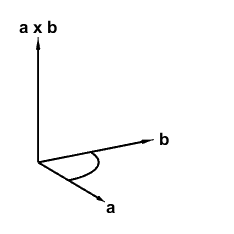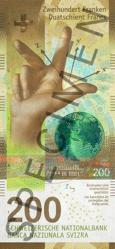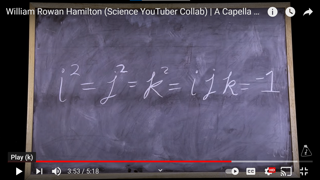# Quaternions

Quaternions are generalizations of the complex numbers that have found applications in computer graphics and many other fields.

### Contents

#### Ed Angel

Ed Angel is my longtime friend from Caltech and New Mexico days. Ed is the author of a popular series of textbooks about computer graphics that has now reached its 8th edition. The books feature the evolution of the OpenGL programming language from Silicon Graphics to WebGL and JavaScript. Ed is working on a MATLAB version and I am kibitzing.

#### Two dimensions

Life in two dimensions is easy. A point in the plane is readily described by its cartesian coordinates, $(x,y)$, or by its polar coordinates, $(r,\theta)$, or by a single complex number $z$. Rotation of the point about the origin is easily described by multiplying the cartesian coordinates by a 2-by-2 orthogonal matrix, or by incrementing the polar angle, or by multiplying $z$ by another complex number.

#### Three dimensions

The situation in three dimensions is more complicated. There are cartesian coordinates $(x,y,z)$, or spherical coordinates $(r,\theta,\psi)$. But what is the three-dimensional analog of the single complex number? Rotations are even more complicated. They require the specification of an axis of rotation as well as the angle. And there are lots of 3-by-3 orthogonal matrices that generate rotations to choose from.

#### William Rowan Hamilton

William Rowan Hamilton (1805-1865) was an Irish mathematician, a professor at Trinity College Dublin, and the Royal Astronomer of Ireland. His name is associated with important concepts throughout mathematics and physics. Like many other mathematicians of his era, he tried unsuccessfully to generalize two-dimensional complex numbers by adding a second complex unit. In 1846 he realized that he actually needed to add three complex units, $i$, $j$ and $k$.

#### Dot and cross products

The key ingredients of Hamilton's quaternion arithmetic are dot and cross products. The dot product, also known as the inner product, is a familiar feature of linear algebra in any number of dimensions. The dot product of two vectors is a scalar.

$$a \cdot b = a_1 b_1 \ + \ a_2 b_2 \ + \ a_3 b_3$$

The cross product is defined only in three dimensions. The cross product of two vectors is a third vector, oriented to be perpendicular to the plane spanned by the first two. If $i$, $j$ and $k$ are unit vectors for the axes in three dimensions, then the cross product of vectors $a$ and $b$ is

$$a \times b = (a_2 b_3 - a_3 b_2) \ i \ + \ (a_3 b_1 - a_1 b_3) \ j \ + \ (a_1 b_2 - a_2 b_1) \ k$$

Here is a snapshot of the cross product.There are two possible orientations for the vector perpendicular to the plane spanned by $a$ and $b$. The conventional choice is made by the right hand rule illustrated on this Swiss bank note and by this emoji on the sign for the Facebook corporate offices.#### Quaternions

A quaternion $q$ has two parts, a scalar $s$ and a vector $v$. The scalar is one real number; think of $s$ as the coefficient of the scalar unit, $1$. The vector consists of three real numbers; they are the coefficients of three imaginary units, $i$, $j$ and $k$. So

$$q = [s,v] = s 1 \ + \ v_1 i \ + v_2 j \ + \ v_3 k$$

Quaternion multiplication is defined so that each of the imaginary units is a square root of $-1$ .

$$i^2 = -1, \ \ j^2 = -1, \ \ k^2 = -1$$

Furthermore, the three units are related to each other by a cyclic property of their products.

$$ij = k, \ \ jk = i, \ \ ki = j$$

These properties imply

$$ji = -k, \ \ kj = -i, \ \ ik = -j$$

Let's call these properties of $i$, $j$ and $k$ under multiplication "Hamilton's Rules".

Suppose we have two quaternions.

$$p = [r,u] = r 1 \ + \ u_1 i \ + u_2 j \ + \ u_3 k$$

$$q = [s,v] = s 1 \ + \ v_1 i \ + v_2 j \ + \ v_3 k$$

$$p + q = [r+s, u+v]$$

#### Quaternion multiplication

Quaternion multiplication is ingenious and complicated.

$$p q = (r + u_1 i + u_2 j + u_3 k)(s + v_1 i + v_2 j + v_3 k)$$

There are four terms in each parentheses, so there are 16 terms in the product. Expand carefully because $i$, $j$ and $k$ do not commute. Apply Hamilton's Rules, $i^2 = -1$, $i j = k$, $j i = -k$, etc. The 16 terms can be arranged like this.

$$p q = [\ r s - (u_1 v_1 + u_2 v_2 + u_3 v_3) \ ,$$

$$\ \ \ \ \ \ \ \ r (v_1 i + v_2 j + v_3 k) + s (u_1 i + u_2 j + u_3 k) \ + (u_2 v_3 - u_3 v_2) i + (u_3 v_1 - u_1 v_3) j + (u_1 v_2 - u_2 v_1) k \ ]$$

We recognize two familiar quantities, the dot and cross products.

$$u \cdot v = u_1 v_1 + u_2 v_2 + u_3 v_3$$

$$u \times v = (u_2 v_3 - u_3 v_2) i + (u_3 v_1 - u_1 v_3) j + (u_1 v_2 - u_2 v_1) k$$

So the result in

$$p q = [r s - u \cdot v, r v + s u + u \times v ]$$

This is Hamilton's definition of the quaternion product.

The units $i$, $j$ and $k$ have scalar parts equal to 0 and vector parts equal to [1,0,0], [0,1,0] and [0,0,1]. Plugging these into the product definition produces, for example,

$$i^2 = [0 -i \cdot i, 0 + 0 + i \times i] = -1$$,

$$i j = [0 - 0, 0 + 0 + i \times j] = k$$

and

$$j i = j \times i = - i \times j = - k$$

#### A Capella Science

A Capella Science is a Patreon YouTube channel. https://www.patreon.com/acapellascience.

In 2016, A Capella Science produced a video featuring two Hamiltons, the immensely popular Broadway musical Hamilton and the Irish mathematician Hamilton. https://www.youtube.com/watch?v=SZXHoWwBcDc. Here is a screen capture of one frame from that video.#### qtn

The 2021a and 2021b releases of MATLAB include a quaternion object that is shared among several of the navigation toolboxes.

Ed and I have our own more basic object, qtn.

   help qtn

  qtn  Basic constructor for quaternions.

qtn(a,b,c,d)
qtn(a,[v1 v2 v3])
qtn(a)

Methods

plus        p + q
minus       p - q
uminus      -q
mtimes      p*q
mldivide    p\q
mrdivide    p/q
mpower      q^n
inv(q)
norm(q)
disp(q)

Functions

rot
Euler
randq
qtn_examples

Notes:
All angles are in degrees.
This object is not vectorized.

Documentation for qtn
doc qtn

Published output in the Help browser
showdemo qtn



The most important qtn method is the quaternion product. I think this code is elegant.

   dbtype 89:96 qtn

89            function r = mtimes(p,q)
90                % p*q
91                p = qtn(p);
92                q = qtn(q);
93                r.s = p.s*q.s - dot(p.v,q.v);
94                r.v = p.s*q.v + q.s*p.v + cross(p.v,q.v);
95                r = qtn(r);
96            end


The entire qtn class definition is only about 150 lines long.

#### basic

Here are a few examples and tests of our basic quaternion object.

Two of the units.

   i = qtn(0,[1 0 0])
j = qtn(0,[0 1 0])

i =

0 + 1i + 0j + 0k

j =

0 + 0i + 1j + 0k


Their product is the third unit.

   k = i*j

k =

0 + 0i + 0j + 1k


All three of these are -1.

   ijk = i*j*k
isq = i^2
ii = i*i

ijk =

-1 + 0i + 0j + 0k

isq =

-1 + 0i + 0j + 0k

ii =

-1 + 0i + 0j + 0k


#### random

Two random qtn's with integer coefficients

   p = randq
q = randq

p =

2 + 8i - 1j + 4k

q =

0 + 4i - 6j + 7k


   p_plus_q = p + q

p_plus_q =

2 + 12i - 7j + 11k


Multiplication.

   pq = p*q

pq =

-66 + 25i - 52j - 30k


Right division.

   r = pq/p

r =

0 + 9.6i + 2j - 2.2k


Left division. p\(p*q/p)*p should be within roundoff error of q.

   test1 = norm(p\r*p - q)

test1 =

4.4409e-16



#### rotations

   type rot

function r = rot(theta, v)
% rot   r = rot(theta,v) is the quaternion (qtn) for
% rotation of angle theta, in degrees, about axis v.
v = v/norm(v);
r = qtn(cosd(theta), sind(theta)*v);
end


A random axis of rotation.

   v = randn(1,3)

v =

-0.1241    1.4897    1.4090



   r = rot(36,v)

r =

0.809017 - 0.0355214i + 0.426247j + 0.403167k


Repeating the 36 degree rotation 10 times is a 360 degree rotation, which should be within roundoff error of the quaternion multiplicative identity.

   e = qtn(1)

test2 = norm(r^10 - e)

e =

1 + 0i + 0j + 0k

test2 =

4.6059e-16



#### Code

(Added, September 11, 2021). You can get the code for qtn and related functions from these links: qtn.m, rot.m, Euler.m, randq.m, qtn_examples_1.m.

Published with MATLAB® R2021b

|

### 댓글

댓글을 남기려면 링크 를 클릭하여 MathWorks 계정에 로그인하거나 계정을 새로 만드십시오.